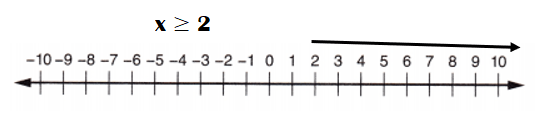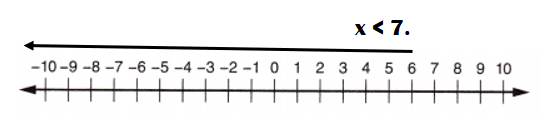Practice questions available in McGraw Hill Math Grade 6 Answer Key PDF Lesson 17.4 Inequalities will engage students and is a great way of informal assessment.

Exercises
Can x be equal to 5?
Question 1.
x – 3 > 4
No, x is not equal to 5 in x – 3 > 4.

Explanation:
x – 3 > 4
=> x > 4 + 3.
=> x > 7.
It means x is greater or equal to 7 but not less than to fulfill the equation.

Question 2.
x + 1 > 3 – 2
No, x is not equal to 5 in x + 1 > 3 – 2.

Explanation:
x + 1 > 3 – 2
=> x + 1 > 1
=> x > 1 – 1
=> x > 0.
It means x is equal or greater to zero but not less than to fulfill the equation.

Question 3.
4 + x ≥ 9
Yes, x is equal to 5 in 4 + x ≥ 9.

Explanation:
4 + x ≥ 9
=> x ≥ 9 – 4
=> x ≥ 5
It means x is equal to 5 and more than yet not less to fulfill the equation.

Question 4.
4x – 1 < 15
No, x is not equal to 5 in 4x – 1 < 15.

Explanation:
4x – 1 < 15
=> 4x < 15 + 1
=> 4x < 16.
=> x < 16 ÷ 4
=> x < 4.
It means x is less than 4 yet not more to fulfill the equation.

Question 5.
x + 12 ≤ 35
Yes, x can be 5 in x + 12 ≤ 35.

Explanation:
x + 12 ≤ 35
=> x  ≤ 35 – 12
=> x  ≤ 23
It means x is less than 24 yet not more to fulfill the equation.

Show the inequality on a number line.
Question 6.

x > 0Explanation:
x > 0
=> x is greater than 0.
=> x = 1, 2, 3,4,5,6,7and so on….

Question 7.
x ≥ 2Explanation:
x ≥ 2
=> x is equal to 2 and greater.
=> x = 2, 3, 4, 5, so on…

Question 8.
x < 7